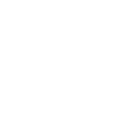×5th Chapter

### 12th Class Mathematics Chapter 5 Test

Here you can prepare 12th Class Mathematics Chapter 5 Linear Inequalities and Linear Programming Test.Click the button for 100% free full practice test.

## 12th class Mathematics chapter 5 online mcq test with answers for Fa part 2 Mathematics Chapter 5 (Linear Inequalities and Linear Programming)

This online test contains MCQs about following topics:.

- Introduction - Linear Inequalities - Region Bounded By 2 or 3 Simultaneous Inequalities - Problem Constraints - Feasible Solution Set - Linear Programming - Linear Programming Problems

FA Part 2 Mathematics Ch 5 Test### 12th class Mathematics chapter 5 online mcq test with answers for Fa part 2 Mathematics Chapter 5 (Linear Inequalities and Linear Programming)

1 A point of a solution region where two of its boundary lines intersects is called a __________ point of the solution region:
• A. Maximum
• B. Corner
• C. Minimum
• D. None of these
2
• A. Open
• B. Closed
• C. Open as well as closed
• D. None of these
3 The feasible region is ________ if it can easily by enclosed within a circle.
• A. Bounded
• B. Exist
• C. Unbounded
• D. None of these
4 The inequality x &lt; a is the open half plane to the __________ of the boundary line x = a:
• A. Above
• B. Left
• C. Below
• D. Right
5
• A. At
• B. Not on
• C. On
• D. None of these
6 The inequality y &gt; b is the open half plane to the ______ of the boundary line y = b:
• A. Above
• B. Left
• C. Below
• D. Right
7 (1, 0) is the solution of inequality :
• A. 7x + 2y &lt; 8
• B. x - 3y &lt; 0
• C. 3x + 5y &gt; 6
• D. -3x + 5y &gt; 2
8 -4 &lt; y &lt; 4 is the solution of the following:
• A. y = 5
• B. y = 3
• C. y = -4
• D. y = 4
9 A line which divides a plane into two parts is called:
• A. Boundary point
• B. Boundary line
• C. Feasible line
• D. None
10 For different values of k, the equation 4x + 5y = k represents lines ______ to the line 4x + 5y = 0.
• A. Perpendicular
• B. Parallel
• C. Equal
• D. None of these

### Top Scorers of FA Part 2 Mathematics Chapter 5 Test Online

H

#### Han Dora

Lahore12 - Jan - 2023

20/20
04 Mins 20 Sec
H

#### Han Dora

Lahore12 - Jan - 2023

18/20
06 Mins 03 Sec#### Saim Maher

Lahore06 - Dec - 2022

17/20
02 Mins 51 Sec
P

#### Panjatan Studio

Lahore09 - Nov - 2022

13/20
04 Mins 53 Sec
H

#### Han Dora

Lahore12 - Jan - 2023

11/20
01 Mins 41 Sec#### Saim Maher

Lahore06 - Dec - 2022

11/20
03 Mins 32 Sec
H

#### Han Dora

Lahore12 - Jan - 2023

10/20
10 Mins 31 Sec

Sort By:
X

to continue to ilmkidunya.comFill the form. Our admission consultants will call you with admission options.

X

to continue to ilmkidunya.com

X

to continue to ilmkidunya.com

X